Products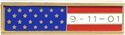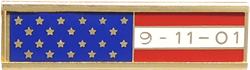A12340

(image does not represent actual size)
Dimensions: 1 3/8" (W) x 5/16" (H)
A12340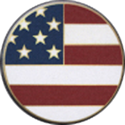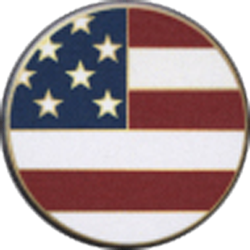A12492

(image does not represent actual size)
Dimensions: 15/16" (D)
A12492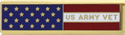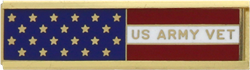A12588

(image does not represent actual size)
Dimensions: 1 3/8" (W) x 5/16" (H)
A12588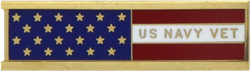A12588-A

(image does not represent actual size)
Dimensions: 1 3/8" (W) x 5/16" (H)
A12588-A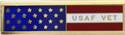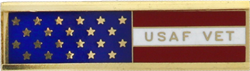A12588-C

(image does not represent actual size)
Dimensions: 1 3/8" (W) x 5/16" (H)
A12588-C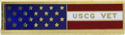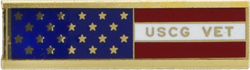A12588-D

(image does not represent actual size)
Dimensions: 1 3/8" (W) x 5/16" (H)
A12588-D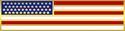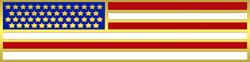A12671

(image does not represent actual size)
Dimensions: 1 1/2" (W) x 3/8" (H)
A12671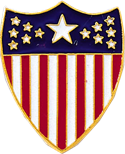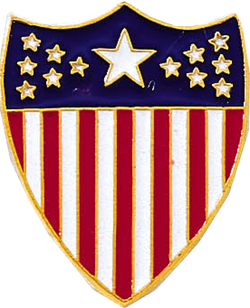A2643

(image does not represent actual size)
Dimensions: 1" (D)
A2643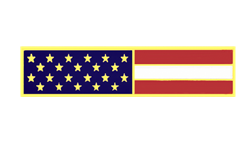A4616-Q

(image does not represent actual size)
Dimensions: 1 3/8" (W) x 5/16" (H)
A4616-Q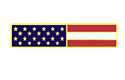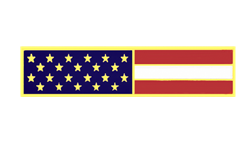A4616-QLS

(image does not represent actual size)
Dimensions: 1 3/8" (W) x 5/16" (H)
A4616-QLS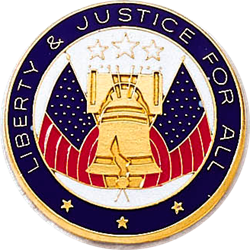A6957

(image does not represent actual size)
Dimensions: 1 1/8" (D)
A6957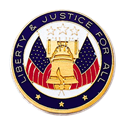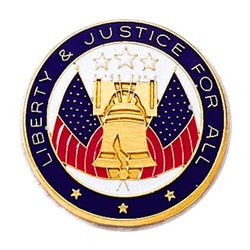A6958

(image does not represent actual size)
Dimensions: 15/16" (D)
A6958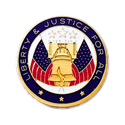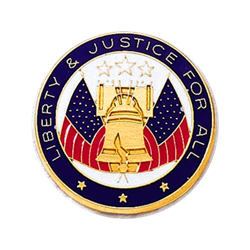A6959

(image does not represent actual size)
Dimensions: 11/16" (D)
A6959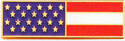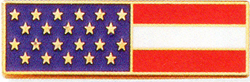A7140-J

(image does not represent actual size)
Dimensions: 1 3/8" (W) x 3/8" (H)
A7140-J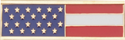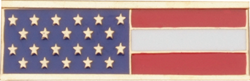A7140-JLS

(image does not represent actual size)
Dimensions: 1 3/8" (W) x 3/8" (H)
A7140-JLS
PRODUCT CATEGORIESAmerican Pride9/11 ProductsBreast CancerEMS/EMTHonor GuardK9Memorial/Remembrance